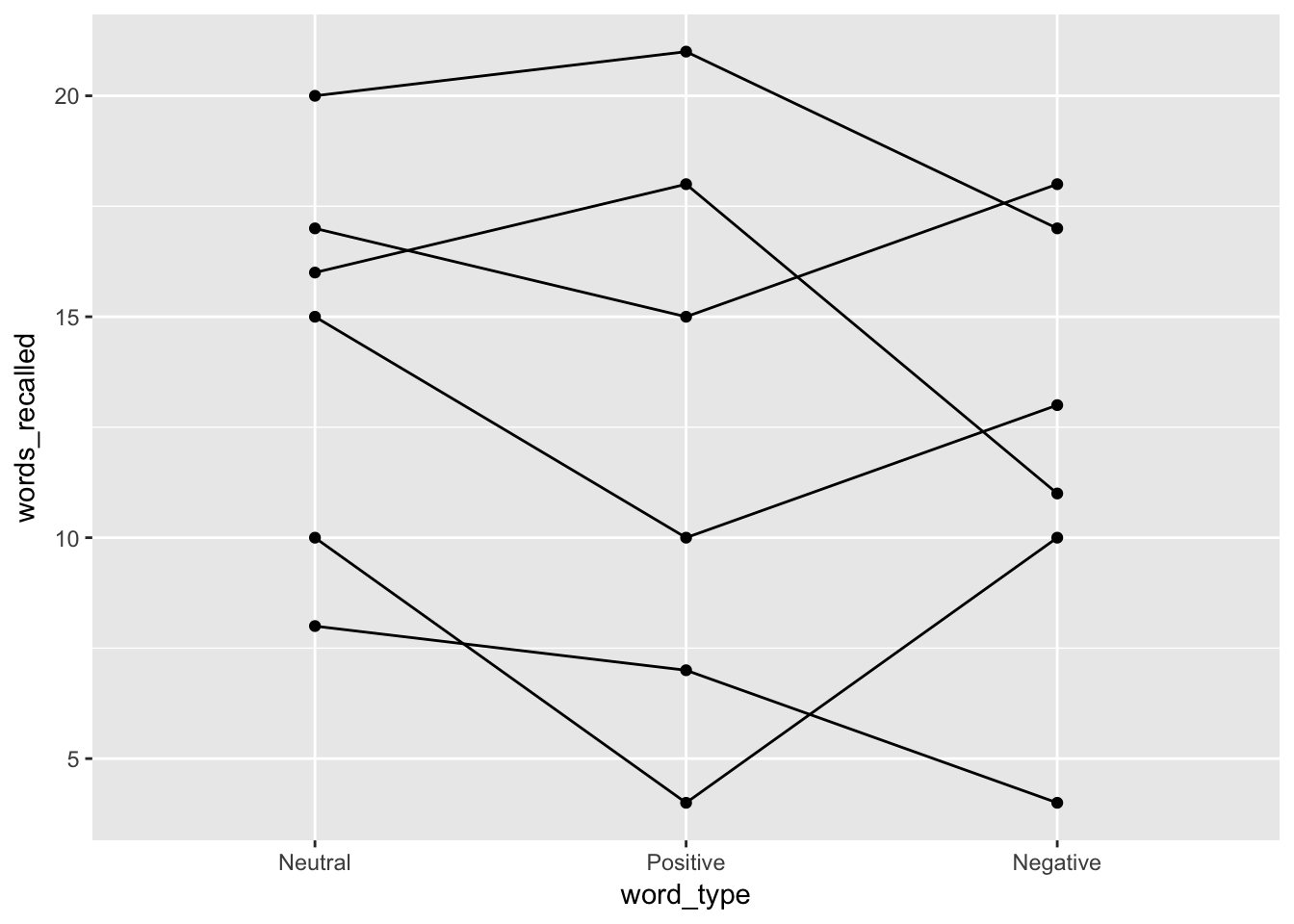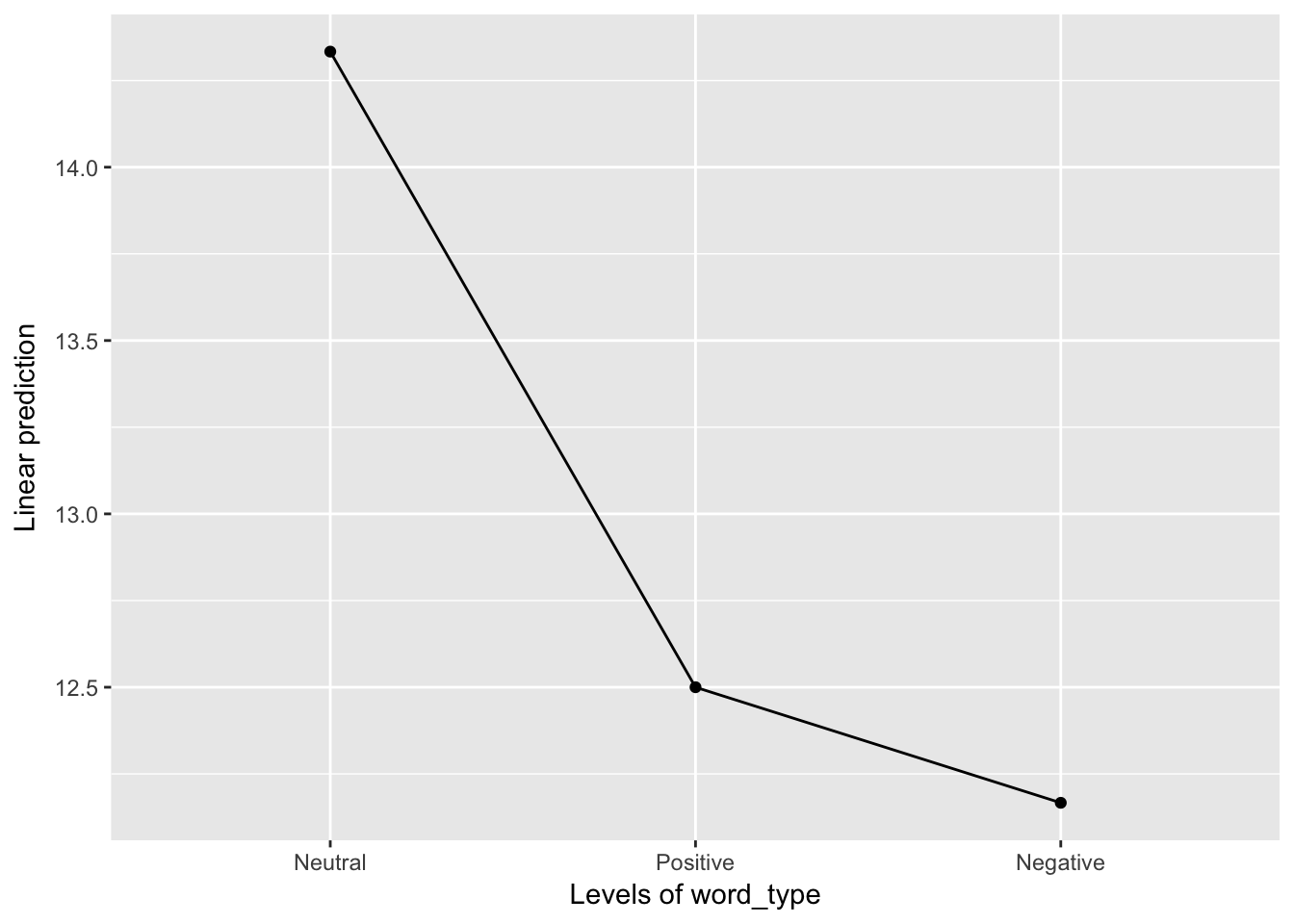## Words Recalled Data Example (Chapter 15a)

### Data Prep

Let’s start with an example of a situation where a mixed ANOVA helps us see patterns that we otherwise miss. To do so, let’s start our R code the same way we usually do, with loading our libraries.

library(tidyverse)
library(furniture)
library(haven)
library(afex)

I input the data as a tribble which saves it as a data.frame and then cleaned up a few of the important variables.

d <- tibble::tribble(
~ID, ~word_type, ~words_recalled,
1,          1,              20,
2,          1,              16,
3,          1,               8,
4,          1,              17,
5,          1,              15,
6,          1,              10,
1,          2,              21,
2,          2,              18,
3,          2,               7,
4,          2,              15,
5,          2,              10,
6,          2,               4,
1,          3,              17,
2,          3,              11,
3,          3,               4,
4,          3,              18,
5,          3,              13,
6,          3,              10) %>%
mutate(word_type = factor(word_type,
labels = c("Neutral", "Positive", "Negative"))) %>%
mutate(fake_id = row_number())
d

### One-Way Independent ANOVA

First, let’s ignore the fact that we know this has repeated measures. As such, we will assume that each word type group is independent. Let’s look at what happens:

ind_anova <- d %>%
afex::aov_4(words_recalled ~ word_type + (1|fake_id),
data = .)
## Contrasts set to contr.sum for the following variables: word_type
ind_anova\$Anova

If we ignored that the word_type groups are not independent, we get an F-statistic = 0.272 and p = 0.765.

What do you think will happen if we account for the repeated measures? Will the F-statistic increase or decrease?

### One-Way RM ANOVA

Now, let’s look at the repeated measures. We do this by using afex::aov_4() and then the summary() functions as shown below.

oneway <- d %>%
afex::aov_4(words_recalled ~ 1 + (word_type|ID),
data = .)
summary(oneway)
##
## Univariate Type III Repeated-Measures ANOVA Assuming Sphericity
##
##              Sum Sq num Df Error SS den Df F value   Pr(>F)
## (Intercept) 3042.00      1   381.33      5 39.8864 0.001466 **
## word_type     16.33      2    68.33     10  1.1951 0.342453
## ---
## Signif. codes:  0 '***' 0.001 '**' 0.01 '*' 0.05 '.' 0.1 ' ' 1
##
##
## Mauchly Tests for Sphericity
##
##           Test statistic  p-value
## word_type         0.2134 0.045538
##
##
## Greenhouse-Geisser and Huynh-Feldt Corrections
##  for Departure from Sphericity
##
##            GG eps Pr(>F[GG])
## word_type 0.55972     0.3282
##
##              HF eps Pr(>F[HF])
## word_type 0.6077293  0.3309148

Here, we see a number of pieces of information, including the sums of squares, F-statistic, and p-value. The F-statistic is now 1.195 and the p-value (although still not signfiicant) is .342. So what happened to the F-statistic? It decreased! So by using the information that these are repeated measures, we have more power. Why is that?

If we look at the output for the two ANOVAs above, both have the sums of squares for word_type at 16.33 so that didn’t change at all. So what did change? Well, it comes down to understanding what is happening to the error term. Although not shown explicitly in the tables above, consider that:

$\text{Independent ANOVA: } SS_{total} = SS_{bet} + SS_w$

$\text{RM ANOVA: } SS_{total} = SS_{RM} + SS_{sub} + SS_{inter}$

$$SS_{total}$$ is the same in both and $$SS_{RM} = SS_{RM}$$ so what we are doing is splitting up the $$SS_w$$ into $$SS_{sub} + SS_{inter}$$ where only the $$SS_{inter}$$ is the error term now.

This means we have more power with the same amount of data.

Let’s now plot this using a spaghetti plot.

d %>%
ggplot(aes(word_type, words_recalled, group = ID)) +
geom_line() +
geom_point()The output provides us with a bit of an understanding of why there is not a significant effect of word_type. In addition to a spaghetti plot, it is often useful to show what the overall repeated measure factor is doing, not the individuals (especially if your sample size is larger than 20). To do that, we can use:

oneway %>%
emmeans::emmip(~ word_type)Although there is a pattern here, we need to consider the scale. Looking at the spaghetti plot, we have individuals that range from 5 to 20 so a difference of 2 or 3 is not large. However, it is clear that a pattern may exist and so we should probably investigate this further, possibly with a larger sample size.